### SQRT Function Examples – Excel & Google Sheets

This Tutorial demonstrates how to use the Excel SQRT Function in Excel to calculate the square root.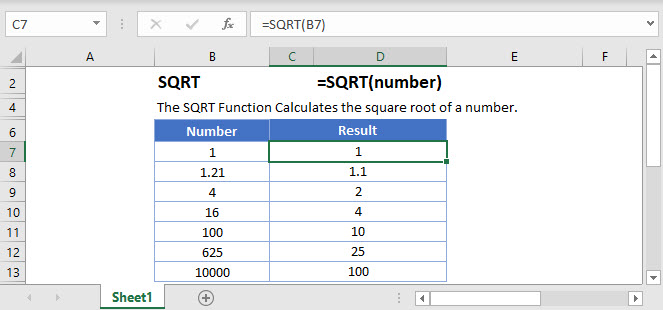## SQRT Function Overview

The SQRT Function Calculates the square root of a number.

To use the SQRT Excel Worksheet Function, select a cell and type: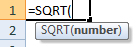(Notice how the formula inputs appear)

## SQRT Function Syntax and Inputs:

number – A number.

AutoMacro - VBA Code Generator

## SQRT Function

The SQRT Function returns the square root of a number.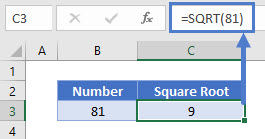The formula calculates the square root of 81. The square root of a number is a value that, when multiplied by itself, gives the number.

## SQRT Function – Negative Number

The SQRT Function returns #NUM! error when negative numbers are entered. The square root of a negative number is undefined.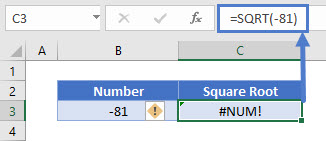## SQRT & ABS Function

You can also calculate the square root of a negative number like its positive, the ABS Function can be used.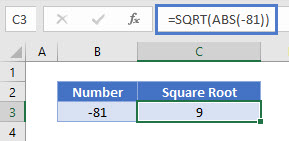Here, the ABS Function converts the negative number into positive number.

## SQRT Function in Google Sheets

The SQRT Function works exactly the same in Google Sheets as in Excel: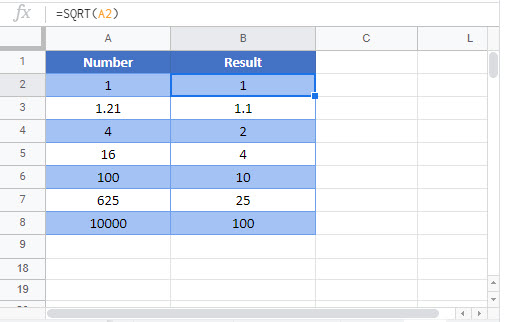## Additional Notes

Use the SQRT Function to calculate the square root of a number.

Alternatively you can raise the number to the .5 power:
`=5^.5 is the same as =sqrt(5) is the same as =product(5,.5)`

Return to the List of all Functions in Excel

## Excel Practice Worksheet

Practice Excel functions and formulas with our 100% free practice worksheets!

• Automatically Graded Exercises
• Learn Excel, Inside Excel!